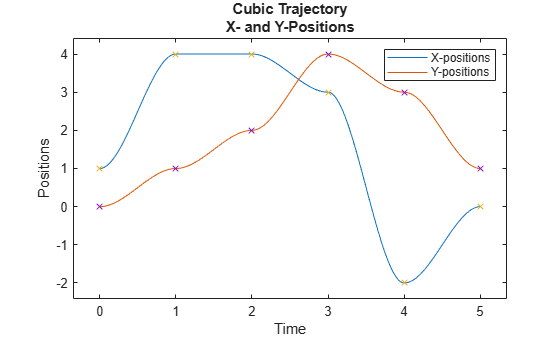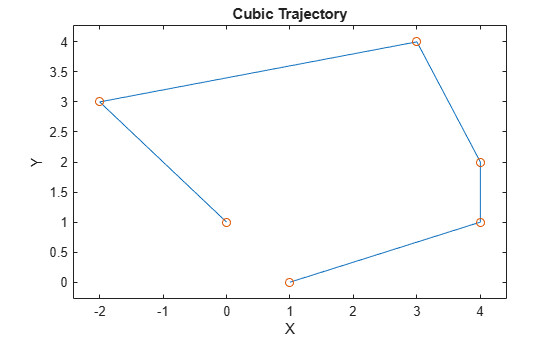# cubicpolytraj

Generate third-order polynomial trajectories

## Syntax

``[q,qd,qdd,pp] = cubicpolytraj(wayPoints,timePoints,tSamples)``
``[q,qd,qdd,pp] = cubicpolytraj(___,Name,Value)``

## Description

example

````[q,qd,qdd,pp] = cubicpolytraj(wayPoints,timePoints,tSamples)` generates a third-order polynomial that achieves a given set of input waypoints with corresponding time points. The function outputs positions, velocities, and accelerations at the given time samples, `tSamples`. The function also returns the piecewise polynomial `pp` form of the polynomial trajectory with respect to time.```
````[q,qd,qdd,pp] = cubicpolytraj(___,Name,Value)` specifies additional parameters as `Name,Value` pair arguments using any combination of the previous syntaxes.```

## Examples

collapse all

Use the `cubicpolytraj` function with a given set of 2-D xy waypoints. Time points for the waypoints are also given.

```wpts = [1 4 4 3 -2 0; 0 1 2 4 3 1]; tpts = 0:5;```

Specify a time vector for sampling the trajectory. Sample at a smaller interval than the specified time points.

`tvec = 0:0.01:5;`

Compute the cubic trajectory. The function outputs the trajectory positions (`q`), velocity (`qd`), acceleration (`qdd`), and polynomial coefficients (`pp`) of the cubic polynomial.

`[q, qd, qdd, pp] = cubicpolytraj(wpts, tpts, tvec);`

Plot the cubic trajectories for the x- and y-positions. Compare the trajectory with each waypoint.

```plot(tvec, q) hold all plot(tpts, wpts, 'x') xlabel('t') ylabel('Positions') legend('X-positions','Y-positions') hold off```You can also verify the actual positions in the 2-D plane. Plot the separate rows of the `q` vector and the waypoints as x- and y -positions.

```figure plot(q(1,:),q(2,:),'-b',wpts(1,:),wpts(2,:),'or') xlabel('X') ylabel('Y')```## Input Arguments

collapse all

Points for waypoints of trajectory, specified as an n-by-p matrix, where n is the dimension of the trajectory and p is the number of waypoints.

Example: `[1 4 4 3 -2 0; 0 1 2 4 3 1]`

Data Types: `single` | `double`

Time points for waypoints of trajectory, specified as a p-element vector.

Example: `[0 2 4 5 8 10]`

Data Types: `single` | `double`

Time samples for the trajectory, specified as an m-element vector. The output position, `q`, velocity, `qd`, and accelerations, `qdd`, are sampled at these time intervals.

Example: `0:0.01:10`

Data Types: `single` | `double`

### Name-Value Pair Arguments

Specify optional comma-separated pairs of `Name,Value` arguments. `Name` is the argument name and `Value` is the corresponding value. `Name` must appear inside quotes. You can specify several name and value pair arguments in any order as `Name1,Value1,...,NameN,ValueN`.

Example: ```'VelocityBoundaryCondition',[1 0 -1 -1 0 0; 1 1 1 -1 -1 -1]```

Velocity boundary conditions for each waypoint, specified as the comma-separated pair consisting of `'VelocityBoundaryCondition'` and an n-by-p matrix. Each row corresponds to the velocity at all p waypoints for the respective variable in the trajectory.

Example: `[1 0 -1 -1 0 0; 1 1 1 -1 -1 -1]`

Data Types: `single` | `double`

## Output Arguments

collapse all

Positions of the trajectory at the given time samples in `tSamples`, returned as an m-element vector, where m is the length of `tSamples`.

Data Types: `single` | `double`

Velocities of the trajectory at the given time samples in `tSamples`, returned as a vector.

Data Types: `single` | `double`

Accelerations of the trajectory at the given time samples in `tSamples`, returned as a vector.

Data Types: `single` | `double`

Piecewise-polynomial, returned as a structure that defines the polynomial for each section of the piecewise trajectory. You can build your own piecewise polynomials using `mkpp`, or evaluate the polynomial at specified times using `ppval`. The structure contains the fields:

• `form`: `'pp'`.

• `breaks`: p-element vector of times when the piecewise trajectory changes forms. p is the number of waypoints.

• `coefs`: n(p–1)-by-`order` matrix for the coefficients for the polynomials. n(p–1) is the dimension of the trajectory times the number of `pieces`. Each set of n rows defines the coefficients for the polynomial that described each variable trajectory.

• `pieces`: p–1. The number of breaks minus 1.

• `order`: Degree of the polynomial + 1. For example, cubic polynomials have an order of 4.

• `dim`: n. The dimension of the control point positions.